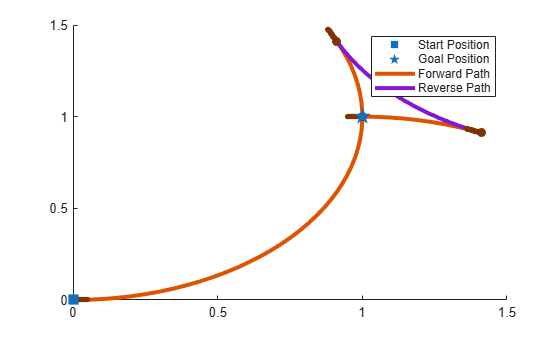# connect

Connect poses for given connection type

## Syntax

``[pathSegments,pathCosts] = connect(connectionObj,start,goal)``
``[pathSegments,pathCosts] = connect(connectionObj,start,goal,'PathSegments','all')``

## Description

example

````[pathSegments,pathCosts] = connect(connectionObj,start,goal)` connects the start and goal poses using the specified `dubinsConnection` object. The path segment object with the lowest cost is returned.```
````[pathSegments,pathCosts] = connect(connectionObj,start,goal,'PathSegments','all')` returns all possible path segments as a cell array with their associated costs.```

## Examples

collapse all

Create a `dubinsConnection` object.

`dubConnObj = dubinsConnection;`

Define start and goal poses as `[x y theta]` vectors.

```startPose = [0 0 0]; goalPose = [1 1 pi];```

Calculate a valid path segment to connect the poses.

`[pathSegObj, pathCosts] = connect(dubConnObj,startPose,goalPose);`

Show the generated path.

`show(pathSegObj{1})`Create a `reedsSheppConnection` object.

`reedsConnObj = reedsSheppConnection;`

Define start and goal poses as `[x y theta]` vectors.

```startPose = [0 0 0]; goalPose = [1 1 pi];```

Calculate a valid path segment to connect the poses.

`[pathSegObj,pathCosts] = connect(reedsConnObj,startPose,goalPose);`

Show the generated path.

`show(pathSegObj{1})`## Input Arguments

collapse all

Path connection type, specified as a `dubinsConnection` or `reedsSheppConnection` object. This object defines the parameters of the connection, including the minimum turning radius of the robot and the valid motion types.

Initial pose of the robot at the start of the path segment, specified as an [x, y, Θ] vector or matrix. Each row of the matrix corresponds to a different start pose.

x and y are in meters. Θ is in radians.

The `connect` function supports:

• Singular start pose with singular goal pose.

• Multiple start pose with singular goal pose.

• Singular start pose with multiple goal pose.

• Multiple start pose with multiple goal pose.

The output `pathSegments` cell array size reflects the singular or multiple pose options.

Goal pose of the robot at the end of the path segment, specified as an [x, y, Θ] vector or matrix. Each row of the matrix corresponds to a different goal pose.

x and y are in meters. Θ is in radians.

The `connect` function supports:

• Singular start pose with singular goal pose.

• Multiple start pose with singular goal pose.

• Singular start pose with multiple goal pose.

• Multiple start pose with multiple goal pose.

The output `pathSegments` cell array size reflects the singular or multiple pose options.

## Output Arguments

collapse all

Path segments, specified as a cell array of objects. The type of object depends on the input `connectionObj`. The size of the cell array depends on whether you use singular or multiple `start` and `goal` poses. By default, the function returns the path with the lowest cost for each `start` and `goal` pose. When call `connect` using the `'PathSegments','all'` name-value pair, the cell array contains all valid path segments between the specified `start` and `goal` poses.

Cost of path segments, specified as a positive numeric scalar, vector, or matrix. Each element of the cost vector or matrix corresponds to a path segment in `pathSegment`. By default, the function returns the path with the lowest cost for each start and goal pose.

Example: `[7.6484,7.5122]`

## Extended Capabilities

### C/C++ Code GenerationGenerate C and C++ code using MATLAB® Coder™.

#### Introduced in R2019b